Select PageMost children struggling with fractions. Parents also buy the Fractions book to understand the book for themselves to better to explain it to them children.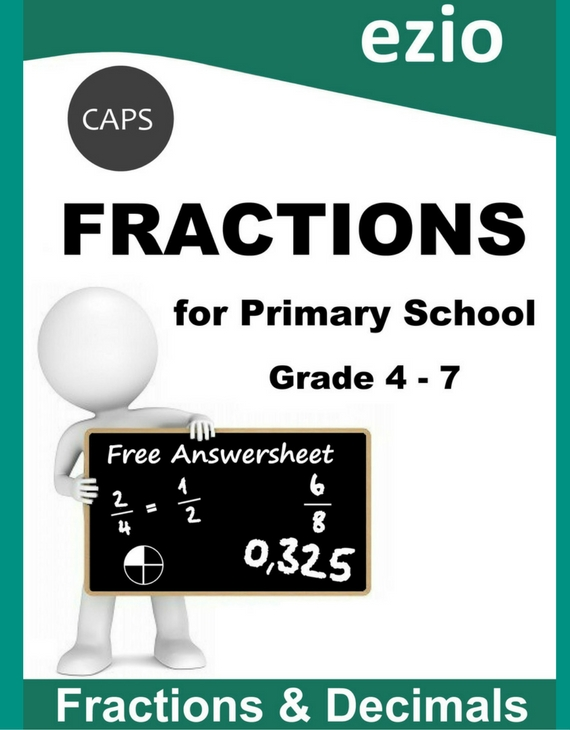Ezio Fractions for Primary School Children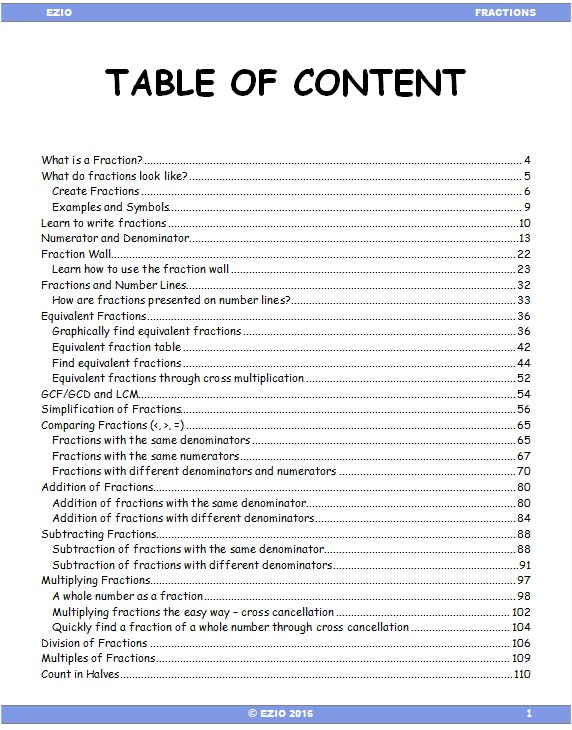What is a Fraction?
Learn to write fractions
Numerator and Denominator
Fraction Wall
Fractions and Number Lines ; Equivalent Fractions
GCF/GCD and LCM; Simplification of Fractions
Comparing Fractions (<, >, =)
Addition of Fractions; Multiplying Fractions
Division of Fractions; Multiples of Fractions
Proper Fractions, Improper Fractions and Mixed Numbers
Decimals; Fractions of a Whole (t, h, th)
Mixed Numbers as Decimals
Adding and Subtracting Decimals
Multiplication and Division of Decimals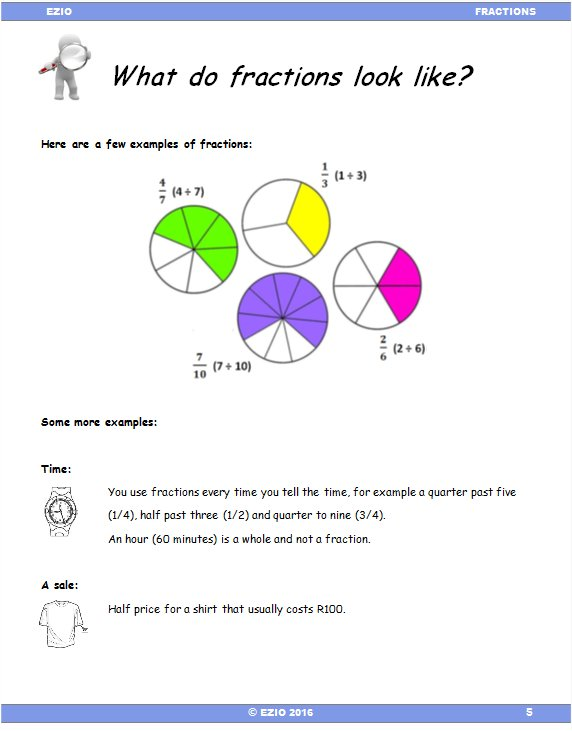Example
What do fractions look like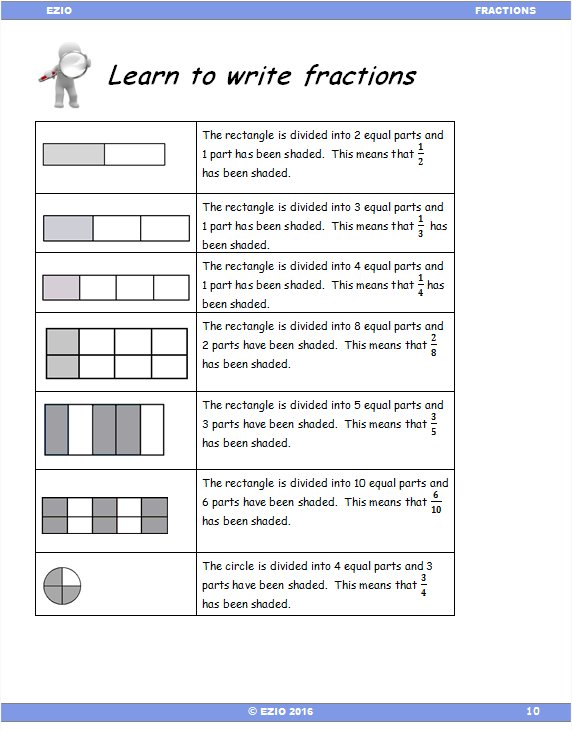Example
Learn to write Fractions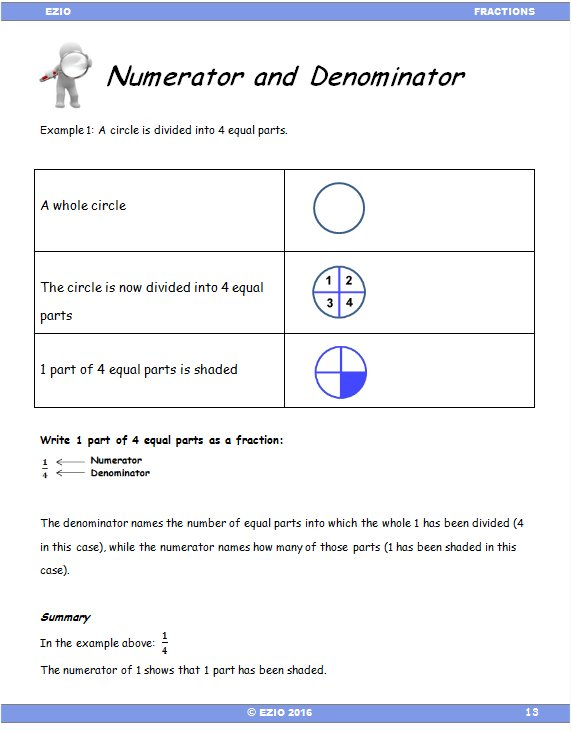Example
Numerator and Denominator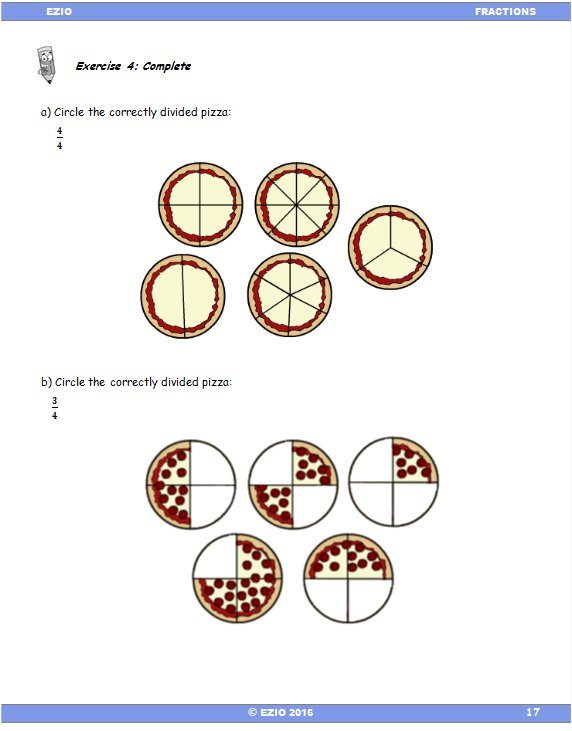Example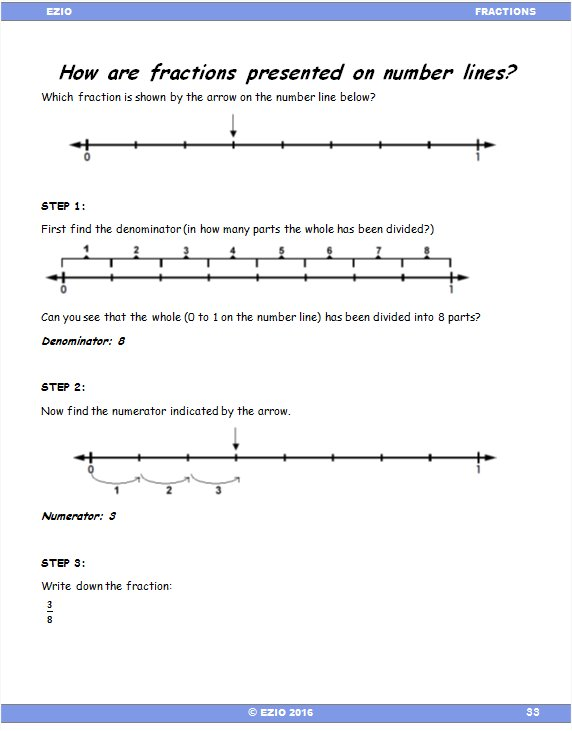Example
How are fractions presented on number lines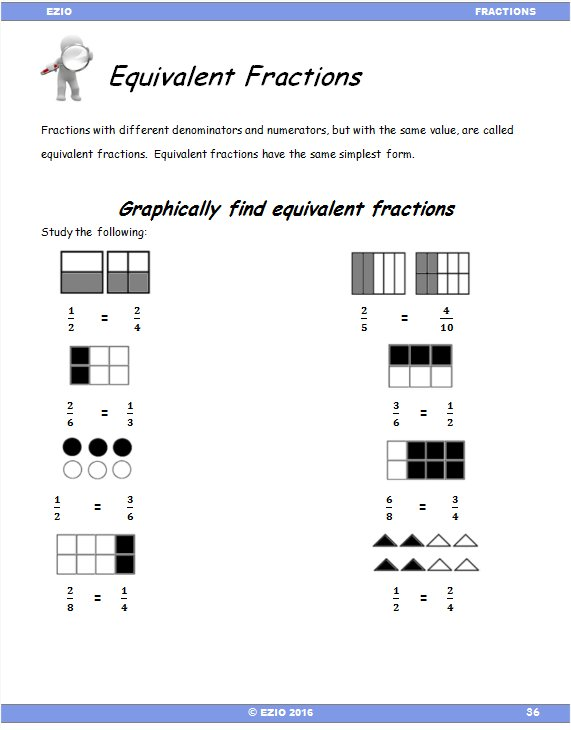Example
Equivalent Fractions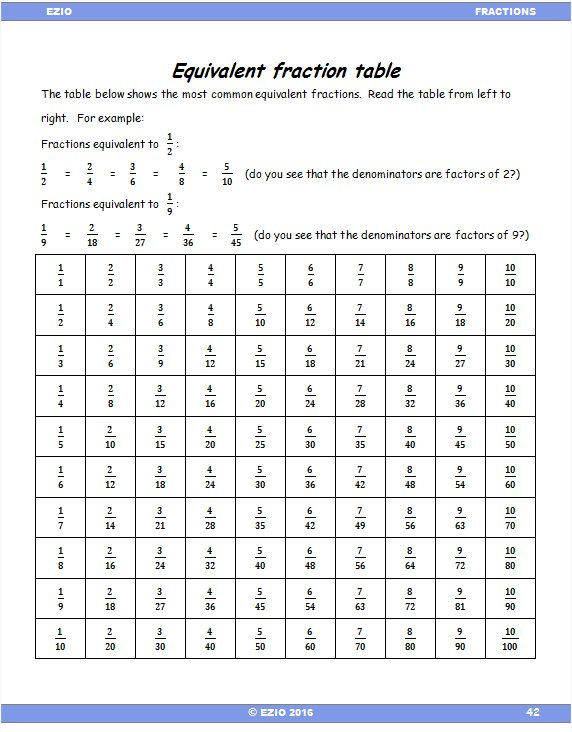Example
Equivalent Fractions Table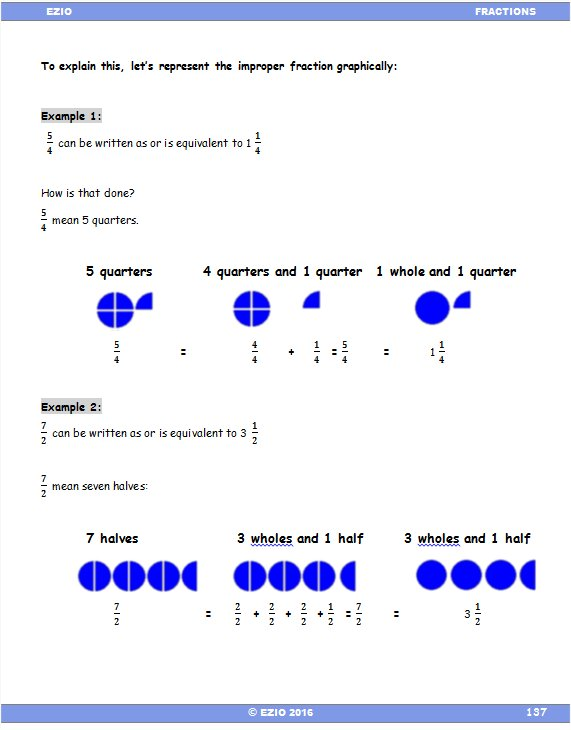Example
Improper Fractions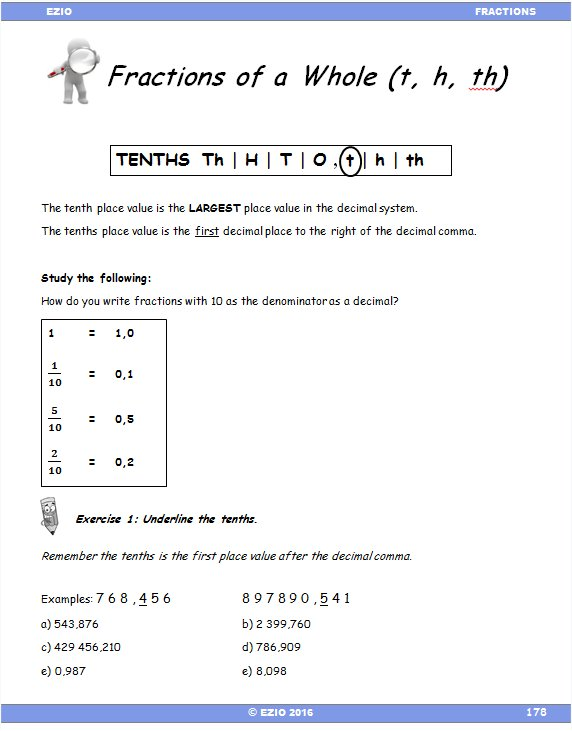Example
Fractions of Whole